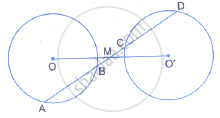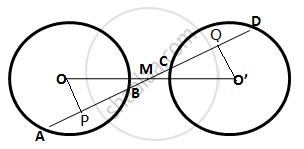Share

A Straight Line is Drawn Cutting Two Equal Circles and Passing Through the Mid-point M of the Line Joining Their Centres O and O’Prove that the Chords Ab and Cd, Which Are Intercepted by the Two - ICSE Class 10 - Mathematics

ConceptChord Properties - Equal Chords Are Equidistant from the Center

Question

A straight line is drawn cutting two equal circles and passing through the mid-point M of the line joining their centres O and O’Prove that the chords AB and CD, which are intercepted by the two circles are equal.

SolutionGiven: A straight line Ad intersects two circles of equal radii at A, B, C and D.

The line joining the centres O O' intersect AD at M
And M is the midpoint of OO'.
To prove: AB = CD
Construction: From O, draw OP⊥AB  and from O’, draw O 'Q⊥CD.

Proof:

In ΔOMP and Δ O' MQ,
∠OMP = ∠O'MQ (vertically opposite angles)
∠OPM = ∠O'QM (each = 90°)
OM = O'M (Given)

By Angle – Angle – Side criterion of congruence,
∴ ΔOMP ≅ O'MQ, (by AAS)

The corresponding parts of the congruent triangle are congruent
∴ OP = O'Q (c.p.ct)

We know that two chords of a circle or equal circles which are equidistant from the centre are equal.
∴ AB = CD

Is there an error in this question or solution?

Video TutorialsVIEW ALL 

Solution A Straight Line is Drawn Cutting Two Equal Circles and Passing Through the Mid-point M of the Line Joining Their Centres O and O’Prove that the Chords Ab and Cd, Which Are Intercepted by the Two Concept: Chord Properties - Equal Chords Are Equidistant from the Center.
S